# Discriminants

Suppose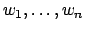are a basis for a number field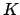, which we view as a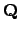-vector space. Let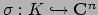be the embedding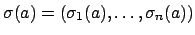, where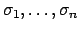are the distinct embeddings ofinto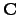. Let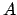be the matrix whose rows are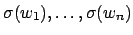. The quantity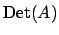depends on the ordering of the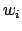, and need not be an integer.

If we consider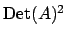instead, we obtain a number that is a well-defined integer which can be either positive or negative. Note that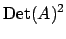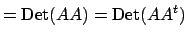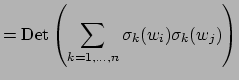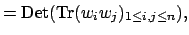socan be defined purely in terms of the trace without mentioning the embeddings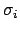. Also, changing the basis for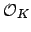is the same as left multiplyingby an integer matrix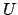of determinant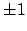, which does not change the squared determinant, since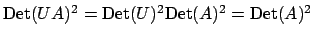. Thusis well defined, and does not depend on the choice of basis.

If we viewas a-vector space, then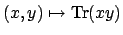defines a bilinear pairing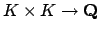on, which we call the . The following lemma asserts that this pairing is nondegenerate, so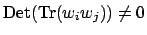hence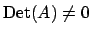.

Lemma 10.2.1   The trace pairing is nondegenerate.

Proof. If the trace pairing is degenerate, then there exists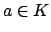such that for every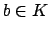we have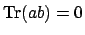. In particularly, taking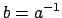we see that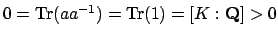, which is absurd.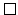Definition 10.2.2 (Discriminant)   Suppose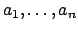is any-basis of. The ofis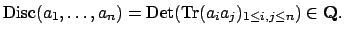The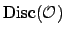of an order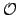inis the discriminant of any basis for. The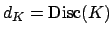of the number fieldis the discrimimant of.

Note that the discriminants defined above are all nonzero by Lemma 10.2.1.

Warning: In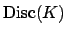is defined to be the discriminant of the polynomial you happened to use to define, which is (in my opinion) a poor choice and goes against most of the literature.

The following proposition asserts that the discriminant of an orderinis bigger than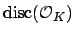by a factor of the square of the index.

Proposition 10.2.3   Supposeis an order in. Then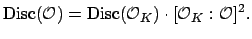Proof. Letbe a matrix whose rows are the images via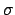of a basis for, and let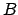be a matrix whose rows are the images viaof a basis for. Since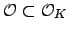has finite index, there is an integer matrix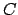such that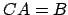, and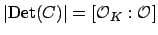. Then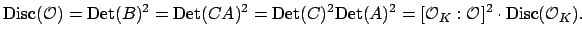This result is enough to give an algorithm for computing, albeit a potentially slow one. Given, find some order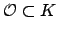, and compute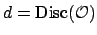. Factor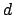, and use the factorization to write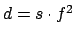, where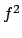is the largest square that divides. Then the index ofinis a divisor of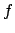, and we (tediously) can enumerate all rings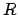with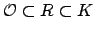and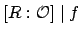, until we find the largest one all of whose elements are integral.

Example 10.2.4   Consider the ring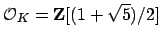of integers of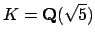. The discriminant of the basis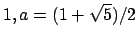is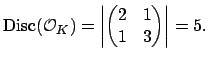Let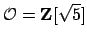be the order generated by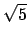. Thenhas basis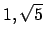, so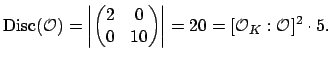William Stein 2004-05-06